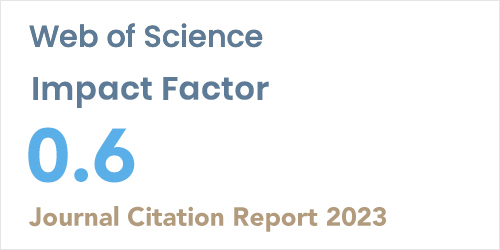ISSN: 1304-7191 | E-ISSN: 1304-7205
Volume: 41 Issue: 4 Year: 2023A sine-cosine wavelet method for the approximation solutions of the fractional Bagley-Torvik equation
1Department of Mathematical Engineering, Yildiz Technical University, Istanbul, Turkey
Sigma J Eng Nat Sci 2022; 40(1): 150-154 DOI: 10.14744/sigma.2021.00033

#### Abstract

Fractional Bagley-Torvik differential equations can be used to model a variety of natural phenomena in many branches of applied mathematics and engineering in general. The focus of this study is on solving the fractional Bagley-Torvik equations by using sine-cosine wavelet. To this end, the operational matrix of fractional integration is obtained for sine-cosine wavelets. By utilizing this matrix, fractional Bagley-Torvik differential equation is transformed to a system of linear algebraic equations with unknown coefficients, which in turn can readily be solved using numerical solution methods. Test examples are provided to demonstrate the validity and efficiency of this approach